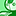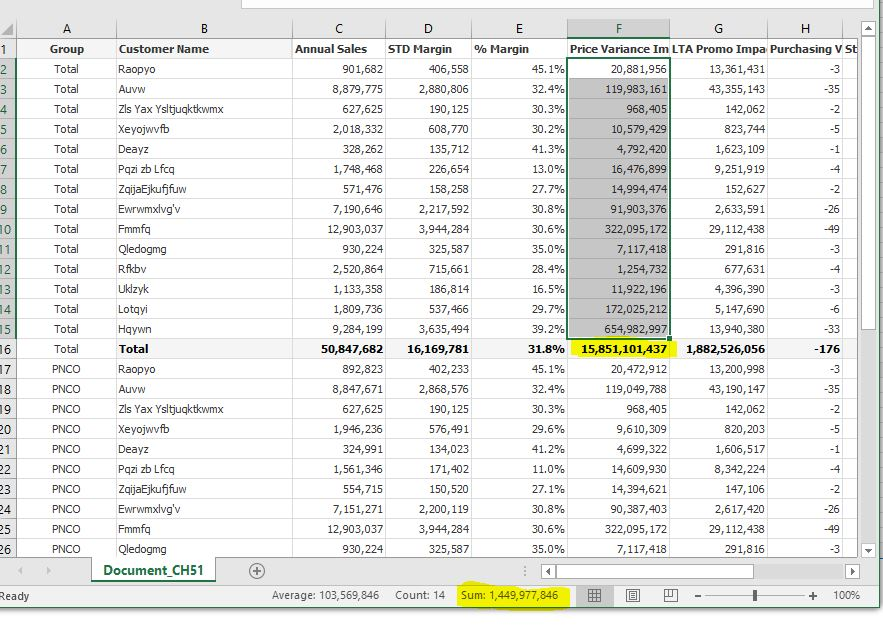# QlikView App Dev

Discussion Board for collaboration related to QlikView App Development.

Announcements
Action-Packed Learning Awaits! QlikWorld 2023. April 17 - 20 in Las Vegas: REGISTER NOW
cancel
Showing results for
Did you mean:Partner - Specialist III

## Additional help with totals in pivot table

Hi all,

I have a pivot table with a pick() dimension that uses a Group Name field and adds in a TOTAL. i have a number of expressions, most of the expressions show the correct total against the total line but there is 1 that don't and i'm unsure why.

the expression that i can't get to total correctly is 'Price Variance Impact', this should show a value of 41,906 against the group name of 'PNCO', the total however is showing 5682 when it should show the same as the 'PNCO' total.

is anyone able to help me understand why?

stalwar1‌ this is something you helped out with initially.

1 Solution

Accepted SolutionsMVP

((sum(aggr((\$(vSTDCostperCase)),Item,[Cust ID], Dim))-

sum(aggr(sum([YTD ACTUAL VALUE])/sum({<Period={"<\$(=GetFieldSelections(Period))"}>}[Sales Volume]),Item, Dim)))

*

\$(vTotalVolumetoLastPeriod))

+

((\$(vSTDCostperCase)-\$(vFutureCost))*\$(vForecastSales))

4 RepliesMVP

((sum(aggr((\$(vSTDCostperCase)),Item,[Cust ID], Dim))-

sum(aggr(sum([YTD ACTUAL VALUE])/sum({<Period={"<\$(=GetFieldSelections(Period))"}>}[Sales Volume]),Item, Dim)))

*

\$(vTotalVolumetoLastPeriod))

+

((\$(vSTDCostperCase)-\$(vFutureCost))*\$(vForecastSales))Partner - Specialist III
Author

Thanks Sunny,

that works for the example i gave, but if i deselect the customer and product they dont match.

looking at each of the customers i can see quite a big difference between them all.

have i done something wrong?Partner - Specialist III
Author

Hi Sunny,

also, i have just exported the table into excel and the total line for the 'Total' group doesnt add up to the sum of rows, i know in a pivot table the total mode is expression tatl, is there a way i can get the total rows instead?

or would i have to look at converting the pivot into a straight table?Partner - Specialist III
Author

Hi Sunny, ignore the last 2 messages, i have fixed it by using

Sum({<Period>} Aggr(alt(sum(aggr(\$(vPriceVarImpact),Item,[Customer Name], Dim)),0), [Item Group Name], [Customer Name], Dim))

in the expression also.

this now works